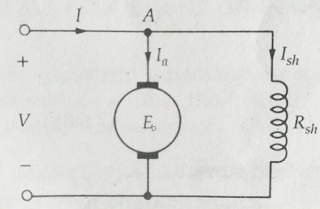DC Shunt Motor | DC shunt motor speed control | DC shunt motor applications - Electrical Diary:Diary of Electrical Engineer -->

DC Shunt Motor | DC shunt motor speed control | DC shunt motor applications

What is DC Shunt Motor?

DC shunt Motors are also self-excited DC Motor. In an electric circuit, if two components are connected in parallel then it is called a shunt. Similar to this, In shunt, the DC Motor field winding is connected in parallel to the armature winding.

Since field winding and armature windings are connected in parallel then applied voltage is the same for both armature and field winding. The connection of Shunt Motors are shown belowDC Shunt Motor

DC Shunt Motor Equation (Relation Between Back EMF and Armature Current)

To study the relation between Back Emf and Armature le consider the simplified circuit diagram given below.Circuit Diagram For DC Shunt Motor
from above-given Circuit diagram
V=is supply voltage
I= Current flowing towards the Motor called input current
Eb = is Induced EMF in armature called Back EMF
Ia =is armature Current
Ish = is field Current called Shunt Current.
Rsh =is net effective resistance of field winding called shunt resistance
From the above-given Circuit diagram, on applying KCL at node A
then we will find that
I=Ia+Ish
on applying KVL we will find that
for field winding
V=IshRsh
and for armature winding the supply voltage will be like this
V=Eb+IaRa

Power Equation for DC Shunt Motor

Power Input to the machine = Mechanical Power Developed + Loss(Armature loss+ Field Winding Loss)
V=Eb+IaRa   ---------(1)
On multiplying By input Current (I) in equation (1)
Then
VI=EbI+I2aRa+ Ish2Rsh
EbI= VI - I2aRa+Ish2Rsh
Developed Mechanical Power = Input Power – Power Loss
Developed Mechanical Power = VI – VIsh –Ia2Ra
Developed Mechanical Power = VI - I2aRa - Ish2Rsh

I2aRa= is armature Loss
Ish2Rsh=is field winding loss

Torque Equation of DC Shunt Motor

Let Developed Torque is T in N-m and Rotor angular Speed is ω radian per second
Developed Mechanical Power = T ω
EbIa = T ω
T= EbIa/ ω

Eb = NPZΦ/60A
After putting the Value Eb of the in above equation, we will get
T = NPZΦIa /60A ω
As we know that ω= 2 πN/60

T= PZΦIa /A 2 π ----------  (2)

From above equation (2)

P= No of Pole,
Z=No of Conductor Coil
Φ = is Flux per
A= No of Parallel Path
Ia= is Armature Current

Out of these all parameter Z, P, and A are constant for given dimension and rating of DC Shunt Motor

Hence after arranging the above equation it may be like this

T = (PZ/2πA)ΦIa
If we will remove PZ/2πA

by a new constant K(say)
Then
T = ΦIa-------(3)
From equation (3)it is clear that the Torque of DC Shunt Motor is Depend on Magnetic Flux and Armature Current.
And also, We know that Flux (Φ) also Depend On the Shunt Branch Current

Φ ∝ Ish

if The Supply terminal Voltage is kept Constant then the Current in the Field Winding (Ish) will Constant then
Φ = Constant

and then Developed Torque will be the Function of only Armature Current
i.e
T∝ Ia

Characteristics of DC Shunt Motors

The relation between two different quantities is represented by a graph and this graphical representation is called the Characteristics and the relation is called the characteristics equation. The variation of independent quantity and its effect on the dependent quantity is shown on the graph.

Torgue-Speed and Speed-Armature characteristics of DC shunt Motors are Similar to Separately excited DC motor and you can look it by clicking here.

Application of DC Shunt Motor

• Lathe Machines
• Centrifugal Pumps
•  Fans, Blowers
• Conveyors, Lifts
• Weaving Machine
• Spinning machines